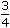# Aptitude - Stocks and Shares

## Why Aptitude Stocks and Shares?

In this section you can learn and practice Aptitude Questions based on "Stocks and Shares" and improve your skills in order to face the interview, competitive examination and various entrance test (CAT, GATE, GRE, MAT, Bank Exam, Railway Exam etc.) with full confidence.

## Where can I get Aptitude Stocks and Shares questions and answers with explanation?

IndiaBIX provides you lots of fully solved Aptitude (Stocks and Shares) questions and answers with Explanation. Solved examples with detailed answer description, explanation are given and it would be easy to understand. All students, freshers can download Aptitude Stocks and Shares quiz questions with answers as PDF files and eBooks.

## Where can I get Aptitude Stocks and Shares Interview Questions and Answers (objective type, multiple choice)?

Here you can find objective type Aptitude Stocks and Shares questions and answers for interview and entrance examination. Multiple choice and true or false type questions are also provided.

## How to solve Aptitude Stocks and Shares problems?

You can easily solve all kind of Aptitude questions based on Stocks and Shares by practicing the objective type exercises given below, also get shortcut methods to solve Aptitude Stocks and Shares problems.

### Exercise :: Stocks and Shares - General Questions

1.

In order to obtain an income of Rs. 650 from 10% stock at Rs. 96, one must make an investment of:

 A. Rs. 3100 B. Rs. 6240 C. Rs. 6500 D. Rs. 9600

Explanation:

To obtain Rs. 10, investment = Rs. 96.

 To obtain Rs. 650, investment = Rs.96 x 650= Rs. 6240. 10

2.

A man bought 20 shares of Rs. 50 at 5 discount, the rate of dividend being 13. The rate of interest obtained is:

A.
 12 1 % 2
B.
 13 1 % 2
C. 15%
D.
 16 2 % 3

Explanation:

Investment = Rs. [20 x (50 - 5)] = Rs. 900.

Face value = Rs. (50 x 20) = Rs. 1000.

 Dividend = Rs.27 x 1000= Rs. 135. 2 100

 Interest obtained =135 x 100% = 15% 900

3.

Which is better investment: 11% stock at 143  or  9% stock at 117?

A. 11% stock at 143
B.
 9 3 % stock at 117 4
C. Both are equally good
D. Cannot be compared, as the total amount of investment is not given.

Explanation:

Let investment in each case be Rs. (143 x 117).

 Income in 1st case = Rs.11 x 143 x 117= Rs. 1287. 143

 Income in 2nd case = Rs.39 x 143 x 117= Rs. 1394.25 4 x 117

 Clearly, 9 3 % stock at 117 is better. 4

4.

A man buys Rs. 20 shares paying 9% dividend. The man wants to have an interest of 12% on his money. The market value of each share is:

 A. Rs. 12 B. Rs. 15 C. Rs. 18 D. Rs. 21

Explanation:

 Dividend on Rs. 20 = Rs.9 x 20= Rs. 9 . 100 5

Rs. 12 is an income on Rs. 100.Rs. 9 is an income on Rs.100 x 9= Rs. 15. 5 12 5

5.

By investing in 16% stock at 64, one earns Rs. 1500. The investment made is:

 A. Rs. 5640 B. Rs. 5760 C. Rs. 7500 D. Rs. 9600

Explanation:

 To earn Rs. 50 , investment = Rs. 64. 3

 To earn Rs. 1500, investment = Rs.64 x 3 x 1500= Rs. 5760. 50